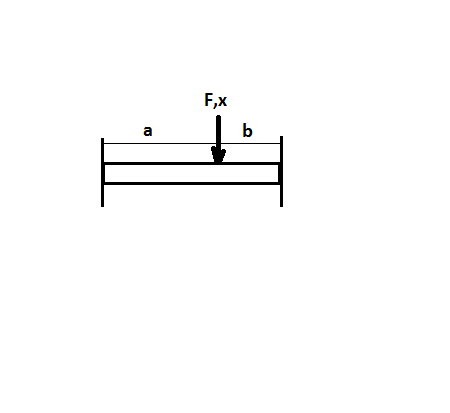# HOW TO FIND SPRING CONSTANT k STEP BY STEP OF THE BEAM ABOVE? Hint: solution for spring constant...

## Question:

HOW TO FIND SPRING CONSTANT k STEP BY STEP OF THE BEAM ABOVE?

Hint: solution for spring constant k is showed in the figure.## Spring constant of beam

Spring Stiffness or spring constant represented by k represents how much resistance it offers to displacement when a force is applied on it.

## Answer and Explanation: 1

Become a Study.com member to unlock this answer! Create your account

For a load acting at an intermediate point(at a distance from one end and b distance from other end) in a fixed beam:

The deflection of the beam due...

See full answer below.

#### Learn more about this topic:Practice Applying Spring Constant Formulas

from

Chapter 17 / Lesson 11
3.4K

In this lesson, you'll have the chance to practice using the spring constant formula. The lesson includes four problems of medium difficulty involving a variety of real-life applications.